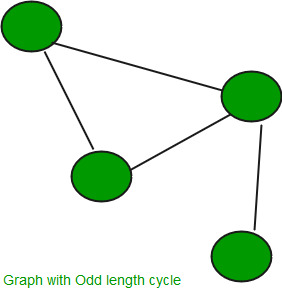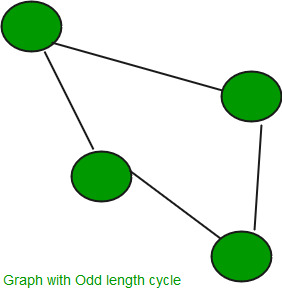# Check if a graphs has a cycle of odd length

• Difficulty Level : Medium
• Last Updated : 07 Nov, 2021

Given a graph, the task is to find if it has a cycle of odd length or not.The idea is based on an important fact that a graph does not contain a cycle of odd length if and only if it is Bipartite, i.e., it can be colored with two colors.
It is obvious that if a graph has an odd length cycle then it cannot be Bipartite. In Bipartite graph there are two sets of vertices such that no vertex in a set is connected with any other vertex of the same set). For a cycle of odd length, two vertices must of the same set be connected which contradicts Bipartite definition.
Let us understand converse, if a graph has no odd cycle then it must be Bipartite. Below is a induction based proof of this taken from http://infohost.nmt.edu/~math/faculty/barefoot/Math321Spring98/BipartiteGraphsAndEvenCycles.html
Assume that (X, Y) is a bipartition of G and let C = u1, u2, . . . , uk be a cycle of G, where u1 is in the vertex set X (abbreviated u1 ∈ X). If u1 ∈X then u2 ∈ Y, . . . and, in general, u2j+1 ∈X and u2i ∈Y. Since C is a cycle, uk ∈Y, so that k = 2s for some positive integer s. Therefore cycle C is even.
Assume that graph G has no odd cycles. It will be shown that such a graph is bipartite. The proof is induction on the number of edges. The assertion is clearly true for a graph with at most one edge. Assume that every graph with no odd cycles and at most q edges is bipartite and let G be a graph with q + 1 edges and with no odd cycles. Let e = uv be an edge of G and consider the graph H = G – uv. By induction, H has a bipartition (X, Y). If e has one end in X and the other end in Y then (X, Y) is a bipartition of G. Hence, assume that u and v are in X. If there were a path, P, between u and v in H then the length of P would be even. Thus, P + uv would be an odd cycle of G. Therefore, u and v must be in lie in different “pieces” or components of H. Thus, we have:where X = X1 & X2 and Y = Y1 ∪ Y2. In this case it is clear that (X1 ∪ Y2, X2 ∪ Y1) is a bipartition of G.Therefore we conclude that every graph with no odd cycles is bipartite. One can construct a bipartition as follows:
(1) Choose an arbitrary vertex x0 and set X0 = {x0}.
(2) Let Y0 be the set of all vertices adjacent to x0 and iterate steps 3-4.
(3) Let Xk be the set of vertices not chosen that are adjacent to a vertex of Yk-1
(4) Let Yk be the set of vertices not chosen that are adjacent to a vertex of Xk-1
(5) If all vertices of G have been chosen then
X = X0 ∪ X1 ∪ X2 ∪. . . and Y = Y0 ∪ Y1 ∪ Y2 ∪ . . .
Below is code to check if a graph has odd cycle or not. The code basically checks whether graph is Bipartite.

## C++

 `// C++ program to find out whether a given graph is``// Bipartite or not``#include ``#define V 4``using` `namespace` `std;``  ` `// This function returns true if graph G[V][V] contains``// odd cycle, else false``bool` `containsOdd(``int` `G[][V], ``int` `src)``{``    ``// Create a color array to store colors assigned ``    ``// to all vertices. Vertex number is used as index ``    ``// in this array. The value '-1' of  colorArr[i] ``    ``// is used to indicate that no color is assigned to``    ``// vertex 'i'.  The value 1 is used to indicate first ``    ``// color is assigned and value 0 indicates second ``    ``// color is assigned.``    ``int` `colorArr[V];``    ``for` `(``int` `i = 0; i < V; ++i)``        ``colorArr[i] = -1;``  ` `    ``// Assign first color to source``    ``colorArr[src] = 1;``  ` `    ``// Create a queue (FIFO) of vertex numbers and ``    ``// enqueue source vertex for BFS traversal``    ``queue <``int``> q;``    ``q.push(src);``  ` `    ``// Run while there are vertices in queue (Similar to BFS)``    ``while` `(!q.empty())``    ``{``        ``// Dequeue a vertex from queue ``        ``int` `u = q.front();``        ``q.pop();``  ` `        ``// Return true if there is a self-loop ``        ``if` `(G[u][u] == 1)``           ``return` `true``;  ``  ` `        ``// Find all non-colored adjacent vertices``        ``for` `(``int` `v = 0; v < V; ++v)``        ``{``            ``// An edge from u to v exists and destination``            ``// v is not colored``            ``if` `(G[u][v] && colorArr[v] == -1)``            ``{``                ``// Assign alternate color to this adjacent``                ``// v of u``                ``colorArr[v] = 1 - colorArr[u];``                ``q.push(v);``            ``}``  ` `            ``// An edge from u to v exists and destination``            ``// v is colored with same color as u``            ``else` `if` `(G[u][v] && colorArr[v] == colorArr[u])``                ``return` `true``;``        ``}``    ``}``  ` `    ``// If we reach here, then all adjacent ``    ``// vertices can be colored with alternate``    ``// color``    ``return` `false``;``}``  ` `// Driver program to test above function``int` `main()``{``    ``int` `G[][V] = {{0, 1, 0, 1},``        ``{1, 0, 1, 0},``        ``{0, 1, 0, 1},``        ``{1, 0, 1, 0}``    ``};``  ` `    ``containsOdd(G, 0) ? cout << ``"Yes"` `: cout << ``"No"``;``    ``return` `0;``}`

## Java

 `// JAVA Code For Check if a graphs has a cycle ``// of odd length``import` `java.util.*;`` ` `class` `GFG {``     ` `    ``public` `static` `int` `V =``4``;``     ` `    ``// This function returns true if graph G[V][V] ``    ``// contains odd cycle, else false``    ``public` `static` `boolean` `containsOdd(``int` `G[][], ``int` `src)``    ``{``        ``// Create a color array to store colors assigned ``        ``// to all vertices. Vertex number is used as ``        ``// index in this array. The value '-1' of ``        ``// colorArr[i] is used to indicate that no color``        ``// is assigned to vertex 'i'.  The value 1 is ``        ``// used to indicate first color is assigned and``        ``// value 0 indicates second color is assigned.``        ``int` `colorArr[] = ``new` `int``[V];``        ``for` `(``int` `i = ``0``; i < V; ++i)``            ``colorArr[i] = -``1``;``       ` `        ``// Assign first color to source``        ``colorArr[src] = ``1``;``       ` `        ``// Create a queue (FIFO) of vertex numbers and ``        ``// enqueue source vertex for BFS traversal``        ``LinkedList q = ``new` `LinkedList();``        ``q.add(src);``       ` `        ``// Run while there are vertices in queue ``        ``// (Similar to BFS)``        ``while` `(!q.isEmpty())``        ``{``            ``// Dequeue a vertex from queue ``            ``int` `u = q.peek();``            ``q.pop();``       ` `            ``// Return true if there is a self-loop ``            ``if` `(G[u][u] == ``1``)``               ``return` `true``;  ``       ` `            ``// Find all non-colored adjacent vertices``            ``for` `(``int` `v = ``0``; v < V; ++v)``            ``{``                ``// An edge from u to v exists and ``                ``// destination v is not colored``                ``if` `(G[u][v] == ``1` `&& colorArr[v] == -``1``)``                ``{``                    ``// Assign alternate color to this ``                    ``// adjacent v of u``                    ``colorArr[v] = ``1` `- colorArr[u];``                    ``q.push(v);``                ``}``       ` `                ``// An edge from u to v exists and ``                ``// destination v is colored with same ``                ``// color as u``                ``else` `if` `(G[u][v] == ``1` `&& colorArr[v] ==``                                          ``colorArr[u])``                    ``return` `true``;``            ``}``        ``}``       ` `        ``// If we reach here, then all adjacent ``        ``// vertices can be colored with alternate``        ``// color``        ``return` `false``;``    ``}``     ` `    ``/* Driver program to test above function */``    ``public` `static` `void` `main(String[] args) ``    ``{`` ` `        ``int` `G[][] = {{``0``, ``1``, ``0``, ``1``},``                     ``{``1``, ``0``, ``1``, ``0``},``                     ``{``0``, ``1``, ``0``, ``1``},``                     ``{``1``, ``0``, ``1``, ``0``}};``                                   ` `           ``if` `(containsOdd(G, ``0``))``               ``System.out.println(``"Yes"``) ;``            ``else``                   ``System.out.println(``"No"``);``    ``}``}``   ` `// This code is contributed by Arnav Kr. Mandal.`

## Python3

 `# Python3 program to find out whether ``# a given graph is Bipartite or not ``import` `queue `` ` `# This function returns true if graph ``# G[V][V] contains odd cycle, else false ``def` `containsOdd(G, src):``    ``global` `V``     ` `    ``# Create a color array to store ``    ``# colors assigned to all vertices.``    ``# Vertex number is used as index ``    ``# in this array. The value '-1' of  ``    ``# colorArr[i] is used to indicate  ``    ``# that no color is assigned to vertex``    ``# 'i'. The value 1 is used to indicate ``    ``# first color is assigned and value 0 ``    ``# indicates second color is assigned. ``    ``colorArr ``=` `[``-``1``] ``*` `V``     ` `    ``# Assign first color to source ``    ``colorArr[src] ``=` `1``     ` `    ``# Create a queue (FIFO) of vertex ``    ``# numbers and enqueue source vertex ``    ``# for BFS traversal ``    ``q ``=` `queue.Queue()``    ``q.put(src) ``     ` `    ``# Run while there are vertices in``    ``# queue (Similar to BFS) ``    ``while` `(``not` `q.empty()):``         ` `        ``# Dequeue a vertex from queue ``        ``u ``=` `q.get()``     ` `        ``# Return true if there is a self-loop ``        ``if` `(G[u][u] ``=``=` `1``): ``            ``return` `True``     ` `        ``# Find all non-colored adjacent vertices ``        ``for` `v ``in` `range``(V):``             ` `            ``# An edge from u to v exists and ``            ``# destination v is not colored ``            ``if` `(G[u][v] ``and` `colorArr[v] ``=``=` `-``1``):``                 ` `                ``# Assign alternate color to this ``                ``# adjacent v of u ``                ``colorArr[v] ``=` `1` `-` `colorArr[u] ``                ``q.put(v)``     ` `            ``# An edge from u to v exists and  ``            ``# destination v is colored with ``            ``# same color as u ``            ``elif` `(G[u][v] ``and` `                  ``colorArr[v] ``=``=` `colorArr[u]): ``                ``return` `True``     ` `    ``# If we reach here, then all ``    ``# adjacent vertices can be ``    ``# colored with alternate color ``    ``return` `False``     ` `# Driver Code``V ``=` `4``G ``=` `[[``0``, ``1``, ``0``, ``1``], ``     ``[``1``, ``0``, ``1``, ``0``], ``     ``[``0``, ``1``, ``0``, ``1``], ``     ``[``1``, ``0``, ``1``, ``0``]]`` ` `if` `containsOdd(G, ``0``):``    ``print``(``"Yes"``)``else``:``    ``print``(``"No"``)`` ` `# This code is contributed by PranchalK`

## C#

 `// C# Code For Check if a graphs has a cycle ``// of odd length ``using` `System;``using` `System.Collections.Generic;`` ` `class` `GFG ``{ ``     ` `    ``public` `static` `int` `V = 4; ``     ` `    ``// This function returns true if graph G[V,V] ``    ``// contains odd cycle, else false ``    ``public` `static` `bool` `containsOdd(``int` `[,]G, ``int` `src) ``    ``{ ``        ``// Create a color array to store colors assigned ``        ``// to all vertices. Vertex number is used as ``        ``// index in this array. The value '-1' of ``        ``// colorArr[i] is used to indicate that no color ``        ``// is assigned to vertex 'i'. The value 1 is ``        ``// used to indicate first color is assigned and ``        ``// value 0 indicates second color is assigned. ``        ``int` `[]colorArr = ``new` `int``[V]; ``        ``for` `(``int` `i = 0; i < V; ++i) ``            ``colorArr[i] = -1; ``         ` `        ``// Assign first color to source ``        ``colorArr[src] = 1; ``         ` `        ``// Create a queue (FIFO) of vertex numbers and ``        ``// enqueue source vertex for BFS traversal ``        ``Queue<``int``> q = ``new` `Queue<``int``>(); ``        ``q.Enqueue(src); ``         ` `        ``// Run while there are vertices in queue ``        ``// (Similar to BFS) ``        ``while` `(q.Count != 0) ``        ``{ ``            ``// Dequeue a vertex from queue ``            ``int` `u = q.Peek(); ``            ``q.Dequeue(); ``         ` `            ``// Return true if there is a self-loop ``            ``if` `(G[u, u] == 1) ``            ``return` `true``; ``         ` `            ``// Find all non-colored adjacent vertices ``            ``for` `(``int` `v = 0; v < V; ++v) ``            ``{ ``                ``// An edge from u to v exists and ``                ``// destination v is not colored ``                ``if` `(G[u, v] == 1 && colorArr[v] == -1) ``                ``{ ``                    ``// Assign alternate color to this ``                    ``// adjacent v of u ``                    ``colorArr[v] = 1 - colorArr[u]; ``                    ``q.Enqueue(v); ``                ``} ``         ` `                ``// An edge from u to v exists and ``                ``// destination v is colored with same ``                ``// color as u ``                ``else` `if` `(G[u,v] == 1 && colorArr[v] == ``                                        ``colorArr[u]) ``                    ``return` `true``; ``            ``} ``        ``} ``         ` `        ``// If we reach here, then all adjacent ``        ``// vertices can be colored with alternate ``        ``// color ``        ``return` `false``; ``    ``} ``     ` `    ``/* Driver code */``    ``public` `static` `void` `Main() ``    ``{ `` ` `        ``int` `[,]G = {{0, 1, 0, 1}, ``                    ``{1, 0, 1, 0}, ``                    ``{0, 1, 0, 1}, ``                    ``{1, 0, 1, 0}}; ``                                     ` `        ``if` `(containsOdd(G, 0)) ``            ``Console.WriteLine(``"Yes"``) ; ``            ``else``                ``Console.WriteLine(``"No"``); ``    ``} ``} ``     ` `// This code has been contributed by 29AjayKumar`

## Javascript

 ``

Output:

`No `

The above algorithm works only if the graph is strongly connected. We can extend it for the cases when graph is not strongly connected (Please refer this for details). In the above code, we always start with source 0 and assume that vertices are visited from it. One important observation is a graph with no edges is also Bipartite. Note that the Bipartite condition says all edges should be from one set to another.
This article is contributed by Kartik. If you like GeeksforGeeks and would like to contribute, you can also write an article using write.geeksforgeeks.org or mail your article to review-team@geeksforgeeks.org. See your article appearing on the GeeksforGeeks main page and help other Geeks.